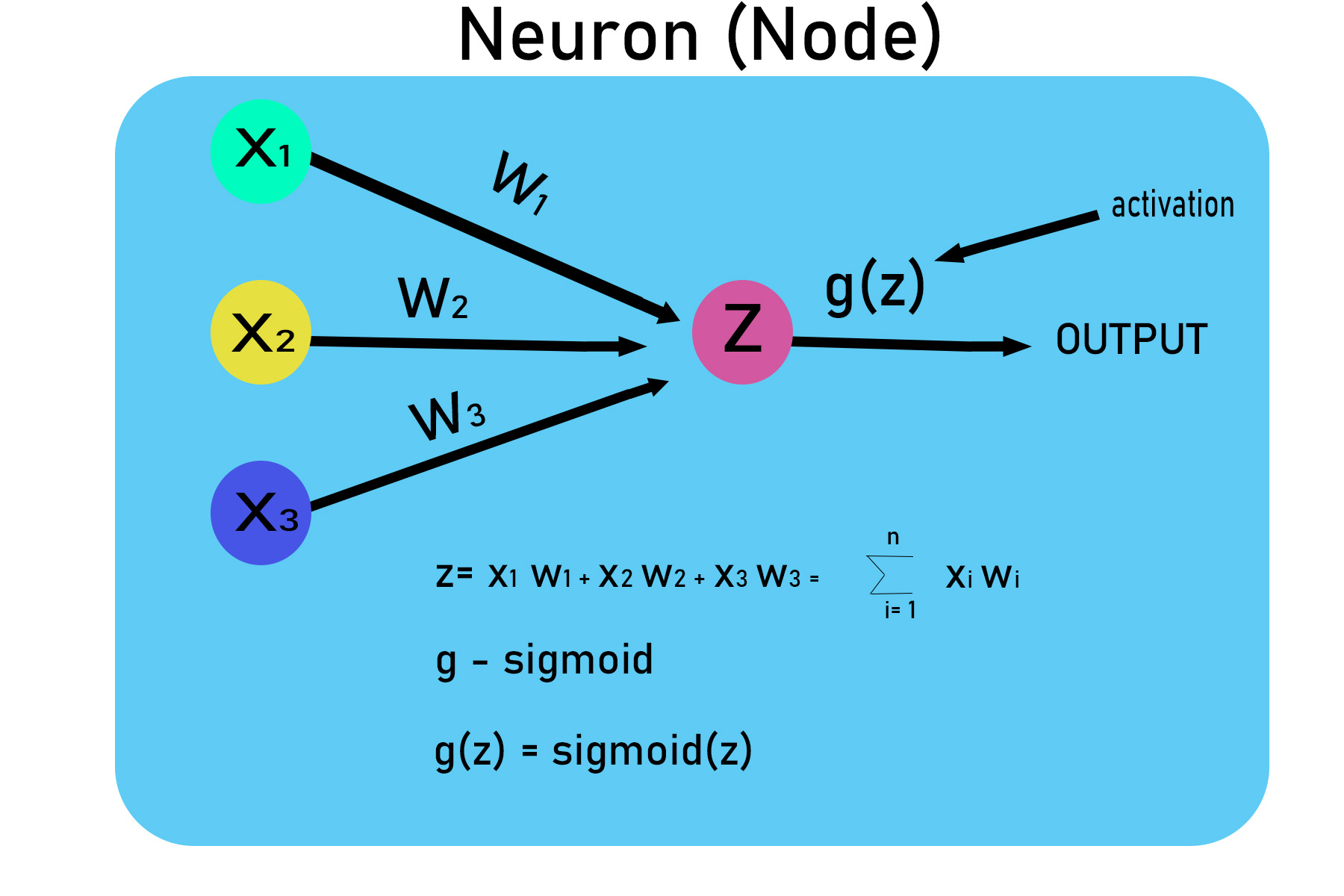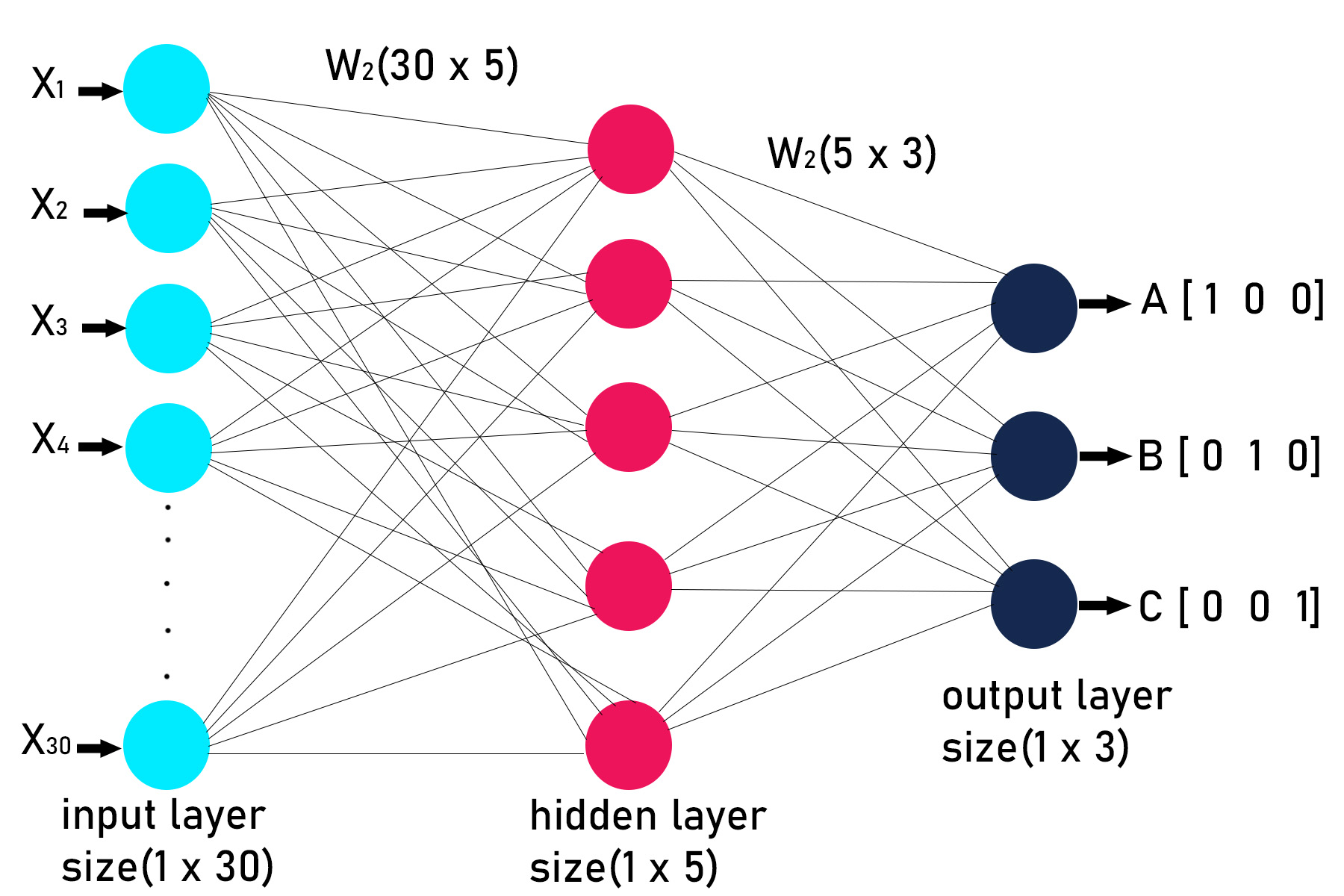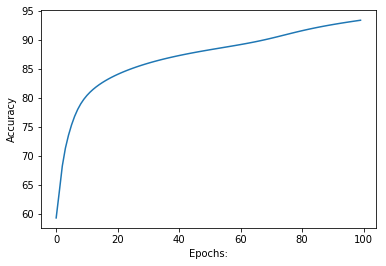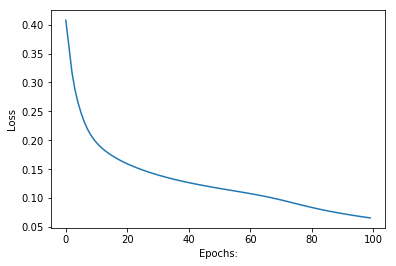# Implementation of neural network from scratch using NumPy

DNN(Deep neural network) in a machine learning algorithm that is inspired by the way the human brain works. DNN is mainly used as a classification algorithm. In this article, we will look at the stepwise approach on how to implement the basic DNN algorithm in NumPy(Python library) from scratch.

The purpose of this article is to create a sense of understanding for the beginners, on how neural network works and   its implementation details. We are going to build a three-letter(A, B, C) classifier, for simplicity   we are going to create the letters (A, B, C) as NumPy array of 0s and 1s, also we are going to ignore the bias term related with each node.Step 1 : Creating the data set using numpy array of 0s and 1s.

As the image is a collection of pixel values in matrix, we will create those matrix of pixel for A, B, C

```using 0 and 1
#A
0 0 1 1 0 0
0 1 0 0 1 0
1 1 1 1 1 1
1 0 0 0 0 1
1 0 0 0 0 1

#B
0 1 1 1 1 0
0 1 0 0 1 0
0 1 1 1 1 0
0 1 0 0 1 0
0 1 1 1 1 0

#C
0 1 1 1 1 0
0 1 0 0 0 0
0 1 0 0 0 0
0 1 0 0 0 0
0 1 1 1 1 0

#Labels for each Letter
A=[1, 0, 0]
B=[0, 1, 0]
C=[0, 0, 1]
```

Code:

 `# Creating data set ` ` `  `# A ` `a ``=``[``0``, ``0``, ``1``, ``1``, ``0``, ``0``, ` `   ``0``, ``1``, ``0``, ``0``, ``1``, ``0``, ` `   ``1``, ``1``, ``1``, ``1``, ``1``, ``1``, ` `   ``1``, ``0``, ``0``, ``0``, ``0``, ``1``, ` `   ``1``, ``0``, ``0``, ``0``, ``0``, ``1``] ` `# B ` `b ``=``[``0``, ``1``, ``1``, ``1``, ``1``, ``0``, ` `   ``0``, ``1``, ``0``, ``0``, ``1``, ``0``, ` `   ``0``, ``1``, ``1``, ``1``, ``1``, ``0``, ` `   ``0``, ``1``, ``0``, ``0``, ``1``, ``0``, ` `   ``0``, ``1``, ``1``, ``1``, ``1``, ``0``] ` `# C ` `c ``=``[``0``, ``1``, ``1``, ``1``, ``1``, ``0``, ` `   ``0``, ``1``, ``0``, ``0``, ``0``, ``0``, ` `   ``0``, ``1``, ``0``, ``0``, ``0``, ``0``, ` `   ``0``, ``1``, ``0``, ``0``, ``0``, ``0``, ` `   ``0``, ``1``, ``1``, ``1``, ``1``, ``0``] ` ` `  `# Creating labels ` `y ``=``[[``1``, ``0``, ``0``], ` `   ``[``0``, ``1``, ``0``], ` `   ``[``0``, ``0``, ``1``]] `

Step 2 : Visualization of data set

 `import` `numpy as np ` `import` `matplotlib.pyplot as plt ` `# visualizing the data, ploting A. ` `plt.imshow(np.array(a).reshape(``5``, ``6``)) ` `plt.show() `

Output:Step 3 :As the data set is in the form of list we will convert it into numpy array.

 `# converting data and labels into numpy array ` ` `  `""" ` `Convert the matrix of 0 and 1 into one hot vector  ` `so that we can directly feed it to the neural network, ` `these vectors are then stored in a list x. ` `"""` ` `  `x ``=``[np.array(a).reshape(``1``, ``30``), np.array(b).reshape(``1``, ``30``),  ` `                                ``np.array(c).reshape(``1``, ``30``)] ` ` `  ` `  `# Labels are also converted into NumPy array ` `y ``=` `np.array(y) ` ` `  ` `  `print``(x, ``"\n\n"``, y)`

Output:

```[array([[0, 0, 1, 1, 0, 0, 0, 1, 0, 0, 1, 0, 1, 1, 1, 1, 1, 1, 1, 0, 0, 0, 0, 1, 1, 0, 0, 0, 0, 1]]),
array([[0, 1, 1, 1, 1, 0, 0, 1, 0, 0, 1, 0, 0, 1, 1, 1, 1, 0, 0, 1, 0, 0, 1, 0, 0, 1, 1, 1, 1, 0]]),
array([[0, 1, 1, 1, 1, 0, 0, 1, 0, 0, 0, 0, 0, 1, 0, 0, 0, 0, 0, 1, 0, 0, 0, 0, 0, 1, 1, 1, 1, 0]])]

[[1 0 0]
[0 1 0]
[0 0 1]]
```

Step 4 : Defining the architecture or structure of the deep neural network. This includes deciding the number of layers and the number of nodes in each layer. Our neural network is going to have the following structure.

```1st layer: Input layer(1, 30)
2nd layer: Hidden layer (1, 5)
3rd layer: Output layer(3, 3)
```Step 5: Declaring and defining all the  function to build deep neural network.

 `# activation function ` ` `  `def` `sigmoid(x): ` `    ``return``(``1``/``(``1` `+` `np.exp(``-``x))) ` `   `  `# Creating the Feed forward neural network ` `# 1 Input layer(1, 30) ` `# 1 hidden layer (1, 5) ` `# 1 output layer(3, 3) ` ` `  `def` `f_forward(x, w1, w2): ` `    ``# hidden ` `    ``z1 ``=` `x.dot(w1)``# input from layer 1  ` `    ``a1 ``=` `sigmoid(z1)``# out put of layer 2  ` `     `  `    ``# Output layer ` `    ``z2 ``=` `a1.dot(w2)``# input of out layer ` `    ``a2 ``=` `sigmoid(z2)``# output of out layer ` `    ``return``(a2) ` `  `  `# initializing the weights randomly ` `def` `generate_wt(x, y): ` `    ``l ``=``[] ` `    ``for` `i ``in` `range``(x ``*` `y): ` `        ``l.append(np.random.randn()) ` `    ``return``(np.array(l).reshape(x, y)) ` `     `  `# for loss we will be using mean square error(MSE) ` `def` `loss(out, Y): ` `    ``s ``=``(np.square(out``-``Y)) ` `    ``s ``=` `np.``sum``(s)``/``len``(y) ` `    ``return``(s) ` `   `  `# Back propagation of error  ` `def` `back_prop(x, y, w1, w2, alpha): ` `     `  `    ``# hiden layer ` `    ``z1 ``=` `x.dot(w1)``# input from layer 1  ` `    ``a1 ``=` `sigmoid(z1)``# output of layer 2  ` `     `  `    ``# Output layer ` `    ``z2 ``=` `a1.dot(w2)``# input of out layer ` `    ``a2 ``=` `sigmoid(z2)``# output of out layer ` `    ``# error in output layer ` `    ``d2 ``=``(a2``-``y) ` `    ``d1 ``=` `np.multiply((w2.dot((d2.transpose()))).transpose(),  ` `                                   ``(np.multiply(a1, ``1``-``a1))) ` ` `  `    ``# Gradient for w1 and w2 ` `    ``w1_adj ``=` `x.transpose().dot(d1) ` `    ``w2_adj ``=` `a1.transpose().dot(d2) ` `     `  `    ``# Updating parameters ` `    ``w1 ``=` `w1``-``(alpha``*``(w1_adj)) ` `    ``w2 ``=` `w2``-``(alpha``*``(w2_adj)) ` `     `  `    ``return``(w1, w2) ` ` `  ` ``def` `train(x, Y, w1, w2, alpha ``=` `0.01``, epoch ``=` `10``): ` `    ``acc ``=``[] ` `    ``losss ``=``[] ` `    ``for` `j ``in` `range``(epoch): ` `        ``l ``=``[] ` `        ``for` `i ``in` `range``(``len``(x)): ` `            ``out ``=` `f_forward(x[i], w1, w2) ` `            ``l.append((loss(out, Y[i]))) ` `            ``w1, w2 ``=` `back_prop(x[i], y[i], w1, w2, alpha) ` `        ``print``(``"epochs:"``, j ``+` `1``, ``"======== acc:"``, (``1``-``(``sum``(l)``/``len``(x)))``*``100``)    ` `        ``acc.append((``1``-``(``sum``(l)``/``len``(x)))``*``100``) ` `        ``losss.append(``sum``(l)``/``len``(x)) ` `    ``return``(acc, losss, w1, w2) ` `  `  `def` `predict(x, w1, w2): ` `    ``Out ``=` `f_forward(x, w1, w2) ` `    ``maxm ``=` `0` `    ``k ``=` `0` `    ``for` `i ``in` `range``(``len``(Out[``0``])): ` `        ``if``(maxm

Step 6: Initializing the weights, as the neural network is having 3 layers, so there will be 2 weight matrix associate with it. The size of each matrix depends on the number of nodes in two connecting layers.
Code:

 `w1 ``=` `generate_wt(``30``, ``5``) ` `w2 ``=` `generate_wt(``5``, ``3``) ` `print``(w1, ``"\n\n"``, w2)`

Output:

```[[ 0.75696605 -0.15959223 -1.43034587  0.17885107 -0.75859483]
[-0.22870119  1.05882236 -0.15880572  0.11692122  0.58621482]
[ 0.13926738  0.72963505  0.36050426  0.79866465 -0.17471235]
[ 1.00708386  0.68803291  0.14110839 -0.7162728   0.69990794]
[-0.90437131  0.63977434 -0.43317212  0.67134205 -0.9316605 ]
[ 0.15860963 -1.17967773 -0.70747245  0.22870289  0.00940404]
[ 1.40511247 -1.29543461  1.41613069 -0.97964787 -2.86220777]
[ 0.66293564 -1.94013093 -0.78189238  1.44904122 -1.81131482]
[ 0.4441061  -0.18751726 -2.58252033  0.23076863  0.12182448]
[-0.60061323  0.39855851 -0.55612255  2.0201934   0.70525187]
[-1.82925367  1.32004437  0.03226202 -0.79073523 -0.20750692]
[-0.25756077 -1.37543232 -0.71369897 -0.13556156 -0.34918718]
[ 0.26048374  2.49871398  1.01139237 -1.73242425 -0.67235417]
[ 0.30351062 -0.45425039 -0.84046541 -0.60435352 -0.06281934]
[ 0.43562048  0.66297676  1.76386981 -1.11794675  2.2012095 ]
[-1.11051533  0.3462945   0.19136933  0.19717914 -1.78323674]
[ 1.1219638  -0.04282422 -0.0142484  -0.73210071 -0.58364205]
[-1.24046375  0.23368434  0.62323707 -1.66265946 -0.87481714]
[ 0.19484897  0.12629217 -1.01575241 -0.47028007 -0.58278292]
[ 0.16703418 -0.50993283 -0.90036661  2.33584006  0.96395524]
[-0.72714199  0.39000914 -1.3215123   0.92744032 -1.44239943]
[-2.30234278 -0.52677889 -0.09759073 -0.63982215 -0.51416013]
[ 1.25338899 -0.58950956 -0.86009159 -0.7752274   2.24655146]
[ 0.07553743 -1.2292084   0.46184872 -0.56390328  0.15901276]
[-0.52090565 -2.42754589 -0.78354152 -0.44405857  1.16228247]
[-1.21805132 -0.40358444 -0.65942185  0.76753095 -0.19664978]
[-1.5866041   1.17100962 -1.50840821 -0.61750557  1.56003127]
[ 1.33045269 -0.85811272  1.88869376  0.79491455 -0.96199293]
[-2.34456987  0.1005953  -0.99376025 -0.94402235 -0.3078695 ]
[ 0.93611909  0.58522915 -0.15553566 -1.03352997 -2.7210093 ]]

[[-0.50650286 -0.41168428 -0.7107231 ]
[ 1.86861492 -0.36446849  0.97721539]
[-0.12792125  0.69578056 -0.6639736 ]
[ 0.58190462 -0.98941614  0.40932723]
[ 0.89758789 -0.49250365 -0.05023684]]

```

Step 7 : Training the model.

 `"""The arguments of train function are data set list x,  ` `correct labels y, weights w1, w2, learning rate = 0.1,  ` `no of epochs or iteration.The function will return the ` `matrix of accuracy and loss and also the matrix of  ` `trained weights w1, w2"""` ` `  `acc, losss, w1, w2 ``=` `train(x, y, w1, w2, ``0.1``, ``100``)`

Output:

```epochs: 1 ======== acc: 59.24962411875523
epochs: 2 ======== acc: 63.68540644266716
epochs: 3 ======== acc: 68.23850165512243
epochs: 4 ======== acc: 71.30325758406262
epochs: 5 ======== acc: 73.52710796040974
epochs: 6 ======== acc: 75.32860090824263
epochs: 7 ======== acc: 76.8094120430158
epochs: 8 ======== acc: 78.00977196942078
epochs: 9 ======== acc: 78.97728263498026
epochs: 10 ======== acc: 79.76587293092753
epochs: 11 ======== acc: 80.42246589416287
epochs: 12 ======== acc: 80.98214842153129
epochs: 13 ======== acc: 81.4695736928823
epochs: 14 ======== acc: 81.90184308791194
epochs: 15 ======== acc: 82.29094665963427
epochs: 16 ======== acc: 82.64546024973251
epochs: 17 ======== acc: 82.97165532985433
epochs: 18 ======== acc: 83.27421706795944
epochs: 19 ======== acc: 83.55671426703763
epochs: 20 ======== acc: 83.82191341206628
epochs: 21 ======== acc: 84.07199359659367
epochs: 22 ======== acc: 84.30869706017322
epochs: 23 ======== acc: 84.53343682891021
epochs: 24 ======== acc: 84.74737503832276
epochs: 25 ======== acc: 84.95148074055622
epochs: 26 ======== acc: 85.1465730591422
epochs: 27 ======== acc: 85.33335370190892
epochs: 28 ======== acc: 85.51243164226796
epochs: 29 ======== acc: 85.68434197894798
epochs: 30 ======== acc: 85.84956043619462
epochs: 31 ======== acc: 86.0085145818298
epochs: 32 ======== acc: 86.16159256503643
epochs: 33 ======== acc: 86.30914997510234
epochs: 34 ======== acc: 86.45151527443966
epochs: 35 ======== acc: 86.58899414916453
epochs: 36 ======== acc: 86.72187303817682
epochs: 37 ======== acc: 86.85042203982091
epochs: 38 ======== acc: 86.97489734865094
epochs: 39 ======== acc: 87.09554333976325
epochs: 40 ======== acc: 87.21259439177474
epochs: 41 ======== acc: 87.32627651970255
epochs: 42 ======== acc: 87.43680887413676
epochs: 43 ======== acc: 87.54440515197342
epochs: 44 ======== acc: 87.64927495564211
epochs: 45 ======== acc: 87.75162513147157
epochs: 46 ======== acc: 87.85166111297174
epochs: 47 ======== acc: 87.94958829083211
epochs: 48 ======== acc: 88.0456134278342
epochs: 49 ======== acc: 88.13994613312185
epochs: 50 ======== acc: 88.2328004057654
epochs: 51 ======== acc: 88.32439625156803
epochs: 52 ======== acc: 88.4149613686817
epochs: 53 ======== acc: 88.5047328856618
epochs: 54 ======== acc: 88.59395911861766
epochs: 55 ======== acc: 88.68290129028868
epochs: 56 ======== acc: 88.77183512103412
epochs: 57 ======== acc: 88.86105215751232
epochs: 58 ======== acc: 88.95086064702116
epochs: 59 ======== acc: 89.04158569269322
epochs: 60 ======== acc: 89.13356833768444
epochs: 61 ======== acc: 89.22716312996127
epochs: 62 ======== acc: 89.32273362510695
epochs: 63 ======== acc: 89.42064521532092
epochs: 64 ======== acc: 89.52125466556964
epochs: 65 ======== acc: 89.62489584606081
epochs: 66 ======== acc: 89.73186143973956
epochs: 67 ======== acc: 89.84238093800867
epochs: 68 ======== acc: 89.95659604815005
epochs: 69 ======== acc: 90.07453567327377
epochs: 70 ======== acc: 90.19609371190103
epochs: 71 ======== acc: 90.32101373021872
epochs: 72 ======== acc: 90.44888465704626
epochs: 73 ======== acc: 90.57915066786961
epochs: 74 ======== acc: 90.7111362751668
epochs: 75 ======== acc: 90.84408471463895
epochs: 76 ======== acc: 90.97720484616241
epochs: 77 ======== acc: 91.10971995033672
epochs: 78 ======== acc: 91.24091164815938
epochs: 79 ======== acc: 91.37015369432306
epochs: 80 ======== acc: 91.49693294991012
epochs: 81 ======== acc: 91.62085750782504
epochs: 82 ======== acc: 91.74165396819595
epochs: 83 ======== acc: 91.8591569057493
epochs: 84 ======== acc: 91.97329371114765
epochs: 85 ======== acc: 92.0840675282122
epochs: 86 ======== acc: 92.19154028777587
epochs: 87 ======== acc: 92.29581711003155
epochs: 88 ======== acc: 92.3970327467751
epochs: 89 ======== acc: 92.49534030435096
epochs: 90 ======== acc: 92.59090221343706
epochs: 91 ======== acc: 92.68388325695001
epochs: 92 ======== acc: 92.77444539437016
epochs: 93 ======== acc: 92.86274409885533
epochs: 94 ======== acc: 92.94892593090393
epochs: 95 ======== acc: 93.03312709510452
epochs: 96 ======== acc: 93.11547275630565
epochs: 97 ======== acc: 93.19607692356153
epochs: 98 ======== acc: 93.27504274176297
epochs: 99 ======== acc: 93.35246306044819
epochs: 100 ======== acc: 93.42842117607569
```

Step 8 : Plotting the  graphs of loss and accuracy with respect to number of epochs(Iteration).

 `import` `matplotlib.pyplot as plt1 ` ` `  `# ploting accuraccy ` `plt1.plot(acc) ` `plt1.ylabel(``'Accuracy'``) ` `plt1.xlabel(``"Epochs:"``) ` `plt1.show() ` ` `  `# plotting Loss ` `plt1.plot(losss) ` `plt1.ylabel(``'Loss'``) ` `plt1.xlabel(``"Epochs:"``) ` `plt1.show()`

Output:`# the trained weigths are ` `print``(w1, ``"\n"``, w2)`

Output:

```[[-0.23769169 -0.1555992   0.81616823  0.1219152  -0.69572168]
[ 0.36399972  0.37509723  1.5474053   0.85900477 -1.14106725]
[ 1.0477069   0.13061485  0.16802893 -1.04450602 -2.76037811]
[-0.83364475 -0.63609797  0.61527206 -0.42998096  0.248886  ]
[ 0.16293725 -0.49443901  0.47638257 -0.89786531 -1.63778409]
[ 0.10750411 -1.74967435  0.03086382  0.9906433  -0.9976104 ]
[ 0.48454172 -0.68739134  0.78150251 -0.1220987   0.68659854]
[-1.53100416 -0.33999119 -1.07657716  0.81305349 -0.79595135]
[ 2.06592829  1.25309796 -2.03200199  0.03984423 -0.76893089]
[-0.08285231 -0.33820853 -1.08239104 -0.22017196 -0.37606984]
[-0.24784192 -0.36731598 -0.58394944 -0.0434036   0.58383408]
[ 0.28121367 -1.84909298 -0.97302413  1.58393025  0.24571332]
[-0.21185018  0.29358204 -0.79433164 -0.20634606 -0.69157617]
[ 0.13666222 -0.31704319  0.03924342  0.54618961 -1.72226768]
[ 1.06043825 -1.02009526 -1.39511479 -0.98141073  0.78304473]
[ 1.44167174 -2.17432498  0.95281672 -0.76748692  1.16231747]
[ 0.25971927 -0.59872416  1.01291689 -1.45200634 -0.72575161]
[-0.27036828 -1.36721091 -0.43858778 -0.78527025 -0.36159359]
[ 0.91786563 -0.97465418  1.26518387 -0.21425247 -0.25097618]
[-0.00964162 -1.05122248 -1.2747124   1.65647842  1.15216675]
[ 2.63067561 -1.3626307   2.44355269 -0.87960091 -0.39903453]
[ 0.30513627 -0.77390359 -0.57135017  0.72661218  1.44234861]
[ 2.49165837 -0.77744044 -0.14062449 -1.6659343   0.27033269]
[ 1.30530805 -0.93488645 -0.66026013 -0.2839123  -1.21397584]
[ 0.41042422  0.20086176 -2.07552916 -0.12391564 -0.67647955]
[ 0.21339152  0.79963834  1.19499535 -2.17004581 -1.03632954]
[-1.2032222   0.46226132 -0.68314898  1.27665578  0.69930683]
[ 0.11239785 -2.19280608  1.36181772 -0.36691734 -0.32239543]
[-1.62958342 -0.55989702  1.62686431  1.59839946 -0.08719492]
[ 1.09518451 -1.9542822  -1.18507834 -0.5537991  -0.28901241]]
[[ 1.52837185 -0.33038873 -3.45127838]
[ 1.0758812  -0.41879112 -1.00548735]
[-3.59476021  0.55176444  1.14839625]
[ 1.07525643 -1.6250444   0.77552561]
[ 0.82785787 -1.79602953  1.15544384]]
```

Step9: Making prediction.

 `""" ` `The predict function will take the following arguments: ` `1) image matrix ` `2) w1 trained weights ` `3) w2 trained weights ` `"""` `predict(x[``1``], w1, w2)`

Output:

```Image is of letter B.
```My Personal Notes arrow_drop_upCheck out this Author's contributed articles.

If you like GeeksforGeeks and would like to contribute, you can also write an article using contribute.geeksforgeeks.org or mail your article to contribute@geeksforgeeks.org. See your article appearing on the GeeksforGeeks main page and help other Geeks.

Please Improve this article if you find anything incorrect by clicking on the "Improve Article" button below.

Article Tags :
Practice Tags :

Be the First to upvote.

Please write to us at contribute@geeksforgeeks.org to report any issue with the above content.